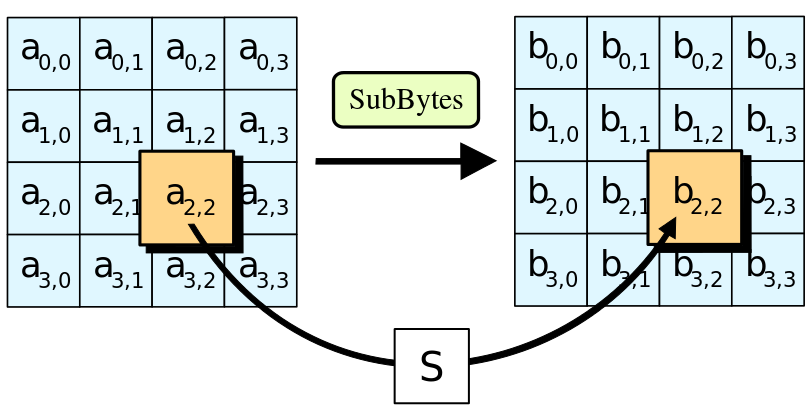# Asymmetric Encryption (RSA)

RSA is one of the first practical public-key cryptosystems and is widely used for secure data transmission. In such acryptosystem, the encryption key is public and differs from the decryption key which is kept secret. In RSA, this asymmetry is based on the practical difficulty of factoring the product of two large prime numbers, the factoring problem. RSA is made of the initial letters of the surnames of Ron Rivest, Adi Shamir, and Leonard Adleman, who first publicly described the algorithm in 1977. Clifford Cocks, an English mathematician, had developed an equivalent system in 1973, but it was notdeclassified until 1997.

A user of RSA creates and then publishes a public key based on two large prime numbers, along with an auxiliary value. The prime numbers must be kept secret. Anyone can use the public key to encrypt a message, but with currently published methods, if the public key is large enough, only someone with knowledge of the prime numbers can feasibly decode the message. Breaking RSA encryption is known as the RSA problem; whether it is as hard as the factoring problem remains an open question.

```public class AsymmetricAlgorithmRSA {

public static final String sTAG = "AsymmetricAlgorithmRSA";

/**
* Generated key pair for 1024-bit RSA encryption and decryption
* @return
*/
public static KeyPair generateKey() {
KeyPair keyPair = null;
try {
KeyPairGenerator kpg = KeyPairGenerator.getInstance("RSA");
kpg.initialize(1024);
keyPair = kpg.genKeyPair();

} catch (Exception e) {
Log.e(sTAG, String.valueOf(R.string.asymmetric_algorithm_rsa_key));
}
return keyPair;
}

/**
* Encoded the original data with RSA private key
* @param privateKey
* @param encryptionText
* @return
*/
public static byte[] encryption(Key privateKey, String encryptionText) {
byte[] encodedBytes = null;
try {
Cipher c = Cipher.getInstance("RSA");
c.init(Cipher.ENCRYPT_MODE, privateKey);
encodedBytes = c.doFinal(encryptionText.getBytes());
} catch (Exception e) {
Log.e(sTAG, String.valueOf(R.string.asymmetric_algorithm_rsa_enryption_error));
}
return encodedBytes;
}

/**
* Decoded the encoded data with RSA public key
* @param publicKey
* @param encodedBytes
* @return
*/
public static byte[] decryption(Key publicKey, byte[] encodedBytes) {
byte[] decodedBytes = null;
try {
Cipher c = Cipher.getInstance("RSA");
c.init(Cipher.DECRYPT_MODE, publicKey);
decodedBytes = c.doFinal(encodedBytes);
} catch (Exception e) {
Log.e(sTAG, String.valueOf(R.string.asymmetric_algorithm_rsa_deryption_error));
}
return decodedBytes;
}
}
```

Example in github

And now in our activity:

```import android.os.Bundle;
import android.support.v7.app.AppCompatActivity;
import android.support.v7.widget.Toolbar;
import android.util.Base64;
import android.view.View;
import android.widget.Button;
import android.widget.EditText;
import android.widget.TextView;

import com.thedeveloperworldisyous.encryptionfile.utils.AsymmetricAlgorithmRSA;
import com.thedeveloperworldisyous.encryptionfile.utils.Utils;

import java.security.Key;
import java.security.KeyPair;

public class RSAActivity extends AppCompatActivity {

public TextView mInPutTextView, mEncodedTextView, mDecoded;
public EditText mEditText;

@Override
protected void onCreate(Bundle savedInstanceState) {
super.onCreate(savedInstanceState);
setContentView(R.layout.activity_rsa);
Toolbar toolbar = (Toolbar) findViewById(R.id.toolbar);
setSupportActionBar(toolbar);

mEditText = (EditText) findViewById(R.id.activity_rsa_edit_text);
mInPutTextView = (TextView)findViewById(R.id.activity_rsa_text_view);
mEncodedTextView  = (TextView)findViewById(R.id.activity_rsa_encode_text_view);
mDecoded = (TextView)findViewById(R.id.activity_rsa_decode_text_view);

setSupportActionBar(toolbar);

}

public void encodeAndDecodeRSA(View view) {

Utils.hideKeyboard(this);
String stringText = mEditText.getText().toString();
mEditText.setText("");
mInPutTextView.setText(stringText);

KeyPair keyPair = AsymmetricAlgorithmRSA.generateKey();
Key publicKey = keyPair.getPublic();
Key privateKey = keyPair.getPrivate();

byte[] encodedBytes = AsymmetricAlgorithmRSA.encryption(privateKey, stringText);
mEncodedTextView.setText(Base64.encodeToString(encodedBytes, Base64.DEFAULT));

byte[] decodedBytes = AsymmetricAlgorithmRSA.decryption(publicKey, encodedBytes);
mDecoded.setText(new String(decodedBytes));
}
}
```

Example in github

The Advanced Encryption Standard (AES), also known as Rijndael (its original name), is a specification for the encryption of electronic data established by the U.S. National Institute of Standards and Technology (NIST) in 2001.AES is based on the Rijndael cipher developed by two Belgian cryptographers, Joan Daemen and Vincent Rijmen, who submitted a proposal to NIST during the AES selection process.Rijndael is a family of ciphers with different key and block sizes.

```public class SymmetricAlgorithmAES {

public static final String sTAG = "SymmetricAlgorithmAES";

public static SecretKeySpec setUpSecrectKey() {
// Set up secret key spec for 128-bit AES encryption and decryption
SecretKeySpec secretKeySpec = null;
try {
SecureRandom sr = SecureRandom.getInstance("SHA1PRNG");
sr.setSeed("any data used as random seed".getBytes());
KeyGenerator kg = KeyGenerator.getInstance("AES");
kg.init(128, sr);
secretKeySpec = new SecretKeySpec((kg.generateKey()).getEncoded(), "AES");
} catch (Exception e) {
Log.e(sTAG, String.valueOf(R.string.symmetric_algorithm_aes_secret_key_error));
}
return secretKeySpec;
}

public static byte[] encryption(SecretKeySpec secretKeySpec, String  theTestText) {
// Encode the original data with AES
byte[] encodedBytes = null;
try {
Cipher c = Cipher.getInstance("AES");
c.init(Cipher.ENCRYPT_MODE, secretKeySpec);
encodedBytes = c.doFinal(theTestText.getBytes());
} catch (Exception e) {
Log.e(sTAG, String.valueOf(R.string.symmetric_algorithm_aes_encryption));
}

return encodedBytes;
}

public static byte[] decryption(SecretKeySpec secretKeySpec, byte[]  encodedBytes) {
// Decode the encoded data with AES
byte[] decodedBytes = null;
try {
Cipher c = Cipher.getInstance("AES");
c.init(Cipher.DECRYPT_MODE, secretKeySpec);
decodedBytes = c.doFinal(encodedBytes);
} catch (Exception e) {
Log.e(sTAG, String.valueOf(R.string.symmetric_algorithm_aes_decryption));
}

return decodedBytes;
}
}
```

Example in GitHub

And now in our activity:

```import android.content.Context;
import android.os.Bundle;
import android.support.v7.app.AppCompatActivity;
import android.support.v7.widget.Toolbar;
import android.util.Base64;
import android.view.View;
import android.view.inputmethod.InputMethodManager;
import android.widget.Button;
import android.widget.EditText;
import android.widget.TextView;

import com.thedeveloperworldisyous.encryptionfile.utils.SymmetricAlgorithmAES;
import com.thedeveloperworldisyous.encryptionfile.utils.Utils;

import javax.crypto.spec.SecretKeySpec;

public class AESActivity extends AppCompatActivity {

public TextView mInPutTextView, mEncodedTextView, mDecoded;
public EditText mEditText;

@Override
protected void onCreate(Bundle savedInstanceState) {
super.onCreate(savedInstanceState);
setContentView(R.layout.activity_aes);
Toolbar toolbar = (Toolbar) findViewById(R.id.toolbar);

mEditText = (EditText) findViewById(R.id.activity_aes_edit_text);
mInPutTextView = (TextView)findViewById(R.id.activity_aes_text_view);
mEncodedTextView  = (TextView)findViewById(R.id.activity_aes_encode_text_view);
mDecoded = (TextView)findViewById(R.id.activity_aes_decode_text_view);

setSupportActionBar(toolbar);
}

public void encodeAndDecodeAES(View view) {
Utils.hideKeyboard(this);
String stringText = mEditText.getText().toString();
mEditText.setText("");
mInPutTextView.setText(stringText);
SecretKeySpec secretKeySpec = SymmetricAlgorithmAES.setUpSecrectKey();

byte[] encodedBytes = SymmetricAlgorithmAES.encryption(secretKeySpec, stringText);
mEncodedTextView.setText(Base64.encodeToString(encodedBytes, Base64.DEFAULT));

byte[] decodedBytes = SymmetricAlgorithmAES.decryption(secretKeySpec, encodedBytes);
mDecoded.setText(new String(decodedBytes));
}
}
```

Example in GitHub